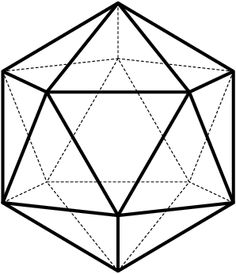# Commemorating the October Revolution!

Probability Level 4Comrade Markov (Андрей Андреевич Марков, 1856-1922) stands at a vertex, $A$, of an icosahedron. After every minute, he travels to one of the five adjacent vertices, along an edge, with equal probability. After 31 hours and 57 minutes (having traveled exactly 1917 times), is he more likely to be back at point $A$ or at the opposite point $B$ of the icosahedron?

Bonus question: After 1917 minutes, is Markov more likely to be at a vertex adjacent to A or at a vertex adjacent to B?

×